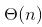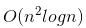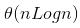Courses

# Sorting MCQ - 1

## 20 Questions MCQ Test RRB JE for Computer Science Engineering | Sorting MCQ - 1

Description
This mock test of Sorting MCQ - 1 for Computer Science Engineering (CSE) helps you for every Computer Science Engineering (CSE) entrance exam. This contains 20 Multiple Choice Questions for Computer Science Engineering (CSE) Sorting MCQ - 1 (mcq) to study with solutions a complete question bank. The solved questions answers in this Sorting MCQ - 1 quiz give you a good mix of easy questions and tough questions. Computer Science Engineering (CSE) students definitely take this Sorting MCQ - 1 exercise for a better result in the exam. You can find other Sorting MCQ - 1 extra questions, long questions & short questions for Computer Science Engineering (CSE) on EduRev as well by searching above.
QUESTION: 1

### What is recurrence for worst case of QuickSort and what is the time complexity in Worst case?

Solution:

The worst case of QuickSort occurs when the picked pivot is always one of the corner elements in sorted array. In worst case, QuickSort recursively calls one subproblem with size 0 and other subproblem with size (n-1). So recurrence is T(n) = T(n-1) + T(0) + O(n) The above expression can be rewritten as T(n) = T(n-1) + O(n) 1

void exchange(int *a, int *b)

int temp;
temp = *a;
*a = *b;
*b = temp;

int partition(int arr[], int si, int ei)

int x = arr[ei];
int i = (si - 1);
int j;

for (j = si; j <= ei - 1; j++)

if(arr[j] <= x)
{
i++;
exchange(&arr[i], &arr[j]);
}

exchange (&arr[i + 1], &arr[ei]);
return (i + 1);

/* Implementation of Quick Sort
arr[] --> Array to be sorted
si --> Starting index
ei --> Ending index
*/
void quickSort(int arr[], int si, int ei)

int pi; /* Partitioning index */
if(si < ei)

pi = partition(arr, si, ei);
quickSort(arr, si, pi - 1);
quickSort(arr, pi + 1, ei);

QUESTION: 2

### Suppose we have a O(n) time algorithm that finds median of an unsorted array. Now consider a QuickSort implementation where we first find median using the above algorithm, then use median as pivot. What will be the worst case time complexity of this modified QuickSort.

Solution:

If we use median as a pivot element, then the recurrence for all cases becomes T(n) = 2T(n/2) + O(n) The above recurrence can be solved using Master Method. It falls in case 2 of master method.

QUESTION: 3

### Which of the following is not a stable sorting algorithm in its typical implementation.

Solution:
QUESTION: 4

Which of the following sorting algorithms in its typical implementation gives best performance when applied on an array which is sorted or almost sorted (maximum 1 or two elements are misplaced).

Solution:

Insertion sort takes linear time when input array is sorted or almost sorted (maximum 1 or 2 elements are misplaced). All other sorting algorithms mentioned above will take more than lienear time in their typical implementation.

QUESTION: 5

Given an unsorted array. The array has this property that every element in array is at most k distance from its position in sorted array where k is a positive integer smaller than size of array. Which sorting algorithm can be easily modified for sorting this array and what is the obtainable time complexity?

Solution:
QUESTION: 6

Consider a situation where swap operation is very costly. Which of the following sorting algorithms should be preferred so that the number of swap operations are minimized in general?

Solution:

Selection sort makes O(n) swaps which is minimum among all sorting algorithms mentioned above.

QUESTION: 7

Which of the following is not true about comparison based sorting algorithms?

Solution:
QUESTION: 8

Suppose we are sorting an array of eight integers using quicksort, and we have just finished the first partitioning with the array looking like this: 2 5 1 7 9 12 11 10 Which statement is correct?

Solution:

7 and 9 both are at their correct positions (as in a sorted array). Also, all elements on left of 7 and 9 are smaller than 7 and 9 respectively and on right are greater than 7 and 9 respectively.

QUESTION: 9

Suppose we are sorting an array of eight integers using heapsort, and we have just finished some heapify (either maxheapify or minheapify) operations. The array now looks like this: 16 14 15 10 12 27 28 How many heapify operations have been performed on root of heap?

Solution:

In Heapsort, we first build a heap, then we do following operations till the heap size becomes 1. a) Swap the root with last element b) Call heapify for root c) reduce the heap size by 1. In this question, it is given that heapify has been called few times and we see that last two elements in given array are the 2 maximum elements in array. So situation is clear, it is maxheapify whic has been called 2 times.

QUESTION: 10

What is the best time complexity of bubble sort?

Solution:

Explanation : The only significant advantage that bubble sort has over most other algorithms, even quicksort, but not insertion sort, is that the ability to detect that the list is sorted efficiently is built into the algorithm. When the list is already sorted (best-case), the complexity of bubble sort is only O(n).

QUESTION: 11

You have to sort 1 GB of data with only 100 MB of available main memory. Which sorting technique will be most appropriate?

Solution:

The data can be sorted using external sorting which uses merging technique. This can be done as follows: 1. Divide the data into 10 groups each of size 100. 2. Sort each group and write them to disk. 3. Load 10 items from each group into main memory. 4. Output the smallest item from the main memory to disk. Load the next item from the group whose item was chosen. 5. Loop step #4 until all items are not outputted. The step 3-5 is called as merging technique.

QUESTION: 12

What is the worst case time complexity of insertion sort where position of the data to be inserted is calculated using binary search?

Solution:

Applying binary search to calculate the position of the data to be inserted doesn't reduce the time complexity of insertion sort. This is because insertion of a data at an appropriate position involves two steps: 1. Calculate the position. 2. Shift the data from the position calculated in step #1 one step right to create a gap where the data will be inserted. Using binary search reduces the time complexity in step #1 from O(N) to O(logN). But, the time complexity in step #2 still remains O(N). So, overall complexity remains O(N^2).

QUESTION: 13

The tightest lower bound on the number of comparisons, in the worst case, for comparison-based sorting is of the order of

Solution:

The number of comparisons that a comparison sort algorithm requires increases in proportion to Nlog(N), where N is the number of elements to sort. This bound is asymptotically tight: Given a list of distinct numbers (we can assume this because this is a worst-case analysis), there are N factorial permutations exactly one of which is the list in sorted order. The sort algorithm must gain enough information from the comparisons to identify the correct permutations. If the algorithm always completes after at most f(N) steps, it cannot distinguish more than 2^f(N) cases because the keys are distinct and each comparison has only two possible outcomes. Therefore, 2^f(N) >= N! or equivalently f(N) >= log(N!). Since log(N!) is Omega(NlogN), the answer is NlogN. For more details, read here

QUESTION: 14

In a modified merge sort, the input array is splitted at a position one-third of the length(N) of the array. What is the worst case time complexity of this merge sort?

Solution:

The time complexity is given by: T(N) = T(N/3) + T(2N/3) + N Solving the above recurrence relation gives, T(N) = N(logN base 3/2)

QUESTION: 15

Which sorting algorithm will take least time when all elements of input array are identical? Consider typical implementations of sorting algorithms.

Solution:

The insertion sort will taketime when input array is already sorted.

QUESTION: 16

A list of n string, each of length n, is sorted into lexicographic order using the merge-sort algorithm. The worst case running time of this computation is

Solution:

The recurrence tree for merge sort will have height Log(n). And O(n2) work will be done at each level of the recurrence tree (Each level involves n comparisons and a comparison takes O(n) time in worst case). So time complexity of this Merge Sort will beQUESTION: 17

In quick sort, for sorting n elements, the (n/4)th smallest element is selected as pivot using an O(n) time algorithm. What is the worst case time complexity of the quick sort?

Solution:

The recursion expression becomes: T(n) = T(n/4) + T(3n/4) + cn After solving the above recursion, we getQUESTION: 18

Consider the Quicksort algorithm. Suppose there is a procedure for finding a pivot element which splits the list into two sub-lists each of which contains at least one-fifth of the elements. Let T(n) be the number of comparisons required to sort n elements. Then

Solution:

For the case where n/5 elements are in one subset, T(n/5) comparisons are needed for the first subset with n/5 elements, T(4n/5) is for the rest 4n/5 elements, and n is for finding the pivot. If there are more than n/5 elements in one set then other set will have less than 4n/5 elements and time complexity will be less than T(n/5) + T(4n/5) + n because recursion tree will be more balanced.

QUESTION: 19

Which of the following sorting algorithms has the lowest worst-case complexity?

Solution:

Worst case complexities for the above sorting algorithms are as follows: Merge Sort — nLogn Bubble Sort — n^2 Quick Sort — n^2 Selection Sort — n^2

QUESTION: 20

Which sorting algorithms is most efficient to sort string consisting of ASCII characters?

Solution:

Counting sort algorithm is efficient when range of data to be sorted is fixed. In the above question, the range is from 0 to 255(ASCII range). Counting sort uses an extra constant space proportional to range of data.